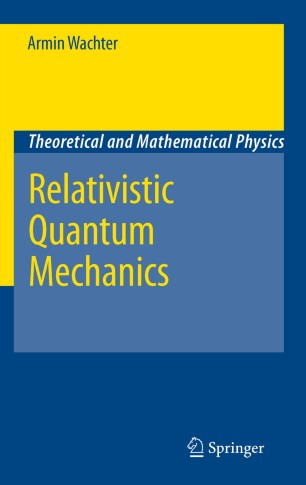# Relativistic Quantum Mechanics

• Armin WachterBook

Part of the Theoretical and Mathematical Physics book series (TMP)

1. Front Matter
Pages i-xvi
2. Armin Wachter
Pages 1-84
3. Armin Wachter
Pages 85-176
4. Armin Wachter
Pages 177-348
5. Back Matter
Pages 349-371

### Introduction

Which problems do arise within relativistic enhancements of the Schrödinger theory, especially if one adheres to the usual one-particle interpretation, and to what extent can these problems be overcome? And what is the physical necessity of quantum field theories? In many books, answers to these fundamental questions are given highly insufficiently by treating the relativistic quantum mechanical one-particle concept very superficially and instead introducing field quantization as soon as possible. By contrast, this monograph emphasizes relativistic quantum mechanics in the narrow sense: it extensively discusses relativistic one-particle concepts and reveals their problems and limitations, therefore motivating the necessity of quantized fields in a physically comprehensible way. The first chapters contain a detailed presentation and comparison of the Klein-Gordon and Dirac theory, always in view of the non-relativistic theory. In the third chapter, we consider relativistic scattering processes and develop the Feynman rules from propagator techniques. This is where the impossibility to get around a quantum field theoretical reasoning is discussed and basic quantum field theoretical concepts are introduced. This book addresses undergraduate and graduate physics students who are interested in a clearly arranged and structured presentation of relativistic quantum mechanics in the "narrow sense" and its connection to quantum field theories. Each section contains a short summary and exercises with solutions. A mathematical appendix rounds up this excellent introductory book on relativistic quantum mechanics.

### Keywords

Dirac equation Dirac theory Feynman propagator Feynman rules Klein-Gordon equation Klein-Gordon theory introducing field quantization limitations of relativistic one-particle quantum mechanics one particle concept one particle quantum mechanics one particle quantum physics one-particle concept quantum field theories quantum field theory quantum mechanics quantum physics relativistic enhancement of Schrödinger theory relativistic one-particle quantum mechanics relativistic one-particle quantum physics relativistic quantum mechanics relativistic scattering process relativistic scattering processes relativistic scattering theory

#### Authors and affiliations

• Armin Wachter
• 1
1. 1.KölnGermany